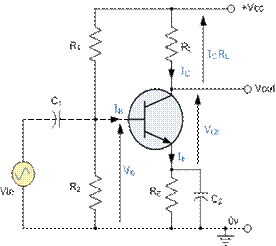EXPT NO:- 2

Title:- Design and simulate a Single stage BJT amplifier, for given specifications.(DC & AC

Analysis)

Objective â€“ To design the single stage CE amplifier and measurement of different parameters such

as gain, input impedance, output impedance and bandwidth.

Pre-requisites:- Biasing of BJT

Instruments & Components:- Computer with Simulation tool Microcap.

Theory:-

Common Emitter Amplifier -

Fig. shows the basic CE circuit with voltage divider biasing. Emitter is at ground potential hence the name common emitter. The signal from the signal source is coupled into the base of transistor through coupling capacitor C1 which provides dc isolation between amplifier and signal source. The dc transistor biasing is established by R1 and R2 and is not disturbed when the signal source is capacitively coupled to amplifier.Consider the common emitter amplifier shown. The resistors provide DC biasing to select an

operating point. The capacitor CE is used to allow the AC to bypass RE.

To perform the design we must first bias the transistor using the curves shown in fig. In

order to work the transistor as an amplifier the operation point (bias point) must be selected at center

CIRCUIT COMPONENTS AND THEIR FUNCTIONS :-

1) Resistors R1,R2 and Re are used for biasing the transistor in the active region, Because to use transistor as an amplifier it is necessary to bias it in active region The type of biasing circuit used here is voltage divider bias

2) RC is collector resistor used for controlling the collector current.

3) Input coupling capacitor C1: the input coupling capacitor C1 is used for Coupling the ac input voltage Vi to the base of transistor as capacitor helps To block any dc component presenting Vi and couples only the ac component of the input single this capacitors also ensures that the dc biasing condition of the transistors remain unchanged even after application of the input signal

4) Bypass capacitor Ce: the capacitor connected in parallel with the emitter resistor Re called as the emitter bypass capacitor. This capacitor offers a low reactance to

amplified ac signal this will increase the voltage gain of the amplifier. Moreover

as CE amplifier acts as open circuit for dc voltage, it dose not bypass R E for dc

conditions. Thus presence of CE does not the dc biasing conditions.

5) Output coupling capacitor C2: this capacitor couples the amplifier output to The

load resistors or to the next stage of amplifier.It is used for blocking the DC part

and passing only the ac part of amplified signal to the load.

FACTORS AFFECTING THE BANDWIDTH OF AMPLIFIER :

1) Coupling capacitors C1 and C2

2) Bypass capacitor Ce.

3) Internal capacitances of the BJT.

EFFECT OF COUPLING CAPACITOR:

1) The capacitor C1 and C2 are coupling capacitors used for blocking the dc Part and allowing only the ac part of the signal to pass through.

2) Reactance of the capacitors is given by, thus the capacitance reactance will Increase with decrease in frequency and vice-versa.Therefore the coupling Capacitors will offer low reactance in mid and high frequency regions and

They can replace by short circuit.

3) A low frequency however the reactance of coupling capacitance will be large. Due to this the voltage drop across them increases with reduction in frequency. This increased voltage drop will reduce both the output and gain of the amplifier in the low frequency region of frequency response.

EFFECT OF BYPASS CAPACITOR:

1) At medium and high frequency the bypass capacitor Ce offers a very low reactance. Therefore it can be replaced by a short circuit. Therefore the impedance ZE which is parallel combination of RE and Xce will have a zero value. Thus RE is bypass successfully and the bypass capacitor CE has no effect on the frequency response of the amplifier at medium and high frequency.

2) But at low frequencies, the reactance Xce is not equal to zero but it has some finite value. Thus the parallel combination of RE and Ce will offer finite impedence.So Re is not properly bypassed.

3) We know that the gain of CE amplifier with bypassed RE is given by

Av = -hfe(RL/Ri)

And the CE amplifier with unbiased RE is given by

Av = -hfe .RL/(hie+ (1+hfe) RE)

4) So if RE is not properly bypassed then the voltage gain will decrease.

Design:- Calculation of Resistors R1, R2, Rc and Re in order to operate transistor in Active

region

1) Consider Vcc = 12Volt , Ic =1mA , VE= 1V , Î²transistor = 100, S=20

With these values of R1 and R2 connect the circuit as shown in fig and simulate it.

Procedure:-

I] DC Analysis :

1) Perform the dynamic DC analysis and observe the node voltages and node currents.

2) Measure VCEQ , VBEQ, ICQ and compare the values with designed values.

II] AC Analysis :

1) Voltage Gain (Av)-

Apply input signal Vs=50mVp-p and measure Vo. Calculate voltage gain Av=Vo/Vs

2) Current Gain (Ai)-

Connect 1k resistor in series with input signal Vs and measure voltage across resistor

Vb and output Voâ€™. Calculate

Ii= (Vs-Vb)/1K Io= Voâ€™/Rc

Ai= Io/Ii

3) Input Impedance (Ri)

Calculate Ri=Voâ€™/(Vo-Voâ€™) X 1k.

4) Output Impedance (Ro)

Disconnect 1K resistor and Connect 10k resistor across the output and measure output Voâ€™â€™. Calculate Ro=[(Vo-Voâ€™â€™)/Voâ€™â€™] X 10k.

5) Calculate Vo/âˆš2 . Measure lower cut of frequency(FL) and upper cut off frequency(FH) at which output falls to Vo/âˆš2. Calculate

Bandwidth = FH - FL

Observations:- Vs=30mVp-p

 Sr. No. Quntity Measured values 1 Vo â€¦â€¦ V 2 Vb â€¦â€¦.V 3 Voâ€™ â€¦â€¦ V 4 Voâ€™â€™ â€¦â€¦.mV 5 FL â€¦ Hz 6 FH â€¦â€¦..Hz

Calculations :

1) Av= Vo/Vs

2) Ri=Voâ€™/(Vo-Voâ€™) X 1k.

3) Ro=(Vo-Voâ€™â€™)/Voâ€™â€™ X 10k.

4) Bandwidth = FH-FL

Result:-

I] DC Analysis -

 Sr. No. Quantity Values Designed Simulated 1 ICQ 2 VCEQ 3 VBEQ

II] AC Analysis -

 Sr. No. Parameter Values 1 Voltage gain (Av) 2 Input Impedance (Ri) 3 Output Impedance (Ro) 4 Bandwidth (B.W.)

Conclusion:- ---------------------------------------------------------------------------------------------------------------------------------------------------------------------------------------------------------------------------------------------------------------------------------------------------------------------------------------------------------------------------------------------------------------------------------------------------------------------------------------------------------------------------------------------------------------------------------------------------------------------------------------------------------------------------------------------------------------------------------------------------------------------------------------------------------------------------------------------------------------------------------------------------------------------------------------------------------------------------------------